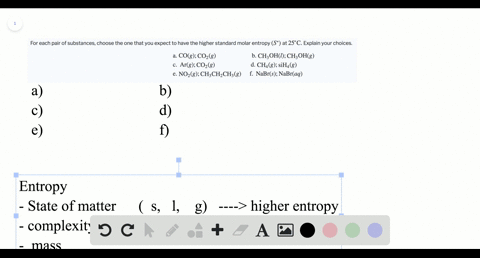Enroll in one of our FREE online STEM summer camps. Space is limited so join now!View Summer Courses### For each pair of substances, choose the one that …

03:42
LP

Need more help? Fill out this quick form to get professional live tutoring.

Get live tutoring
Problem 50

What is the molar entropy of a pure crystal at 0 K? What is the significance of the answer to this question?

The molar entropy of a pure crystal at 0 $\mathrm{K}$ is 0 $\mathrm{J} / \mathrm{mol}$ . K. This is significant
because it allows us to measure all entropy values against the absolute zero
of entropy as defined by the third law.

## Discussion

You must be signed in to discuss.

## Video Transcript

So this question is asking what the Mueller and should be of her of a crystal at zero Kelvin iss. And so Well, basically, I feel glasses. And so you configure. I asked by using the Sportsman equation, which is K l n Tell you, and Kay is a constant and then W's number of states this system can be. And so when you're at zero Kelvin a crystal can only occupy can only be arranged in one way. So w equals one in this case. So entropy now equals K ln one which equals zero. So the sickness of the entropy of ah Crystal at zero Cohen is zero. And so the significance of this is we now have an absolute zero. We can compare all our values too. We don't have to making arbitrary relative value. To compare things to defend will be. We have firm absolute zero that everything else could be compared to, which is useful when calculating all these numbers. So ask the significance of the entropy being 00 k